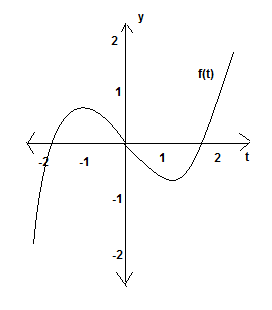# Let F(x) = integral_1^x f(t) d t, where f is the function whose graph is shown. Where is F...

## Question:

Let {eq}\displaystyle F(x) = \int\limits_1^x f(t) d t, {/eq} where {eq}f {/eq} is the function whose graph is shown. Where is {eq}F {/eq} concave downward? Explain.## Derivatives and Antiderivatives

If a function is defined as the integral of another function, that other function is the derivative of the resulting integration. Thus, information about the integrand can be analyzed in the same way that we would analyze information about a derivative.

## Answer and Explanation:

Based on how this function is defined, {eq}f(x) {/eq} is the derivative of {eq}F(x) {/eq}, thanks to the Fundamental Theorem of Calculus. Thus, we can treat the graph given as the graph of the derivative of the function we wish to find information about.

While finding concavity is generally tied to the second derivative, we can actually find when a function is concave up or down using the first derivative as well. This is because the function is concave down when the second derivative is negative, and the second derivative is negative when the first derivative is decreasing. Therefore, we can find the interval on which the function is concave down by finding the interval where the derivative is decreasing.

The function is thus concave down on the interval {eq}(-1, 1) {/eq}, since the graph given is decreasing on that interval.

#### Learn more about this topic:Understanding Concavity and Inflection Points with Differentiation

from Math 104: Calculus

Chapter 10 / Lesson 6
14K